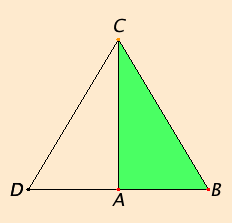# Proposition 48

If in a triangle the square on one of the sides equals the sum of the squares on the remaining two sides of the triangle, then the angle contained by the remaining two sides of the triangle is right.

In the triangle ABC let the square on one side BC equal the sum of the squares on the sides BA and AC

I say that the angle BAC is right.Draw AD from the point A at right angles to the straight line AC. Make AD equal to BA, and join DC.

Since DA equals AB, therefore the square on DA also equals the square on AB.

C.N.2

Add the square on AC to each. Then the sum of the squares on DA and AC equals the sum of the squares on BA and AC.

But the square on DC equals the sum of the squares on DA and AC, for the angle DAC is right, and the square on BC equals the sum of the squares on BA and AC, for this is the hypothesis, therefore the square on DC equals the square on BC, so that the side DC also equals BC.

I.8

Since DA equals AB, and AC is common, the two sides DA and AC equal the two sides BA and AC, and the base DC equals the base BC, therefore the angle DAC equals the angle BAC. But the angle DAC is right, therefore the angle BAC is also right.

Therefore if in a triangle the square on one of the sides equals the sum of the squares on the remaining two sides of the triangle, then the angle contained by the remaining two sides of the triangle is right.

Q.E.D.

## Guide

This proposition is the converse of the previous. It is used in proposition XI.35.